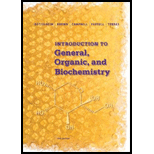# 14-62 Draw structural formulas and write IUPAC names for the eight isomeric alcohols with the molecular formula C 5 H 1 2 O.### Introduction to General, Organic a...

11th Edition
Frederick A. Bettelheim + 4 others
Publisher: Cengage Learning
ISBN: 9781285869759### Introduction to General, Organic a...

11th Edition
Frederick A. Bettelheim + 4 others
Publisher: Cengage Learning
ISBN: 9781285869759

#### Solutions

Chapter
Section
Chapter 14, Problem 14.62P
Textbook Problem

## 14-62 Draw structural formulas and write IUPAC names for the eight isomeric alcohols with the molecular formula C5H12O.

Expert Solution
Interpretation Introduction

Interpretation:

The structures and IUPAC name of all the eight isomeric alcohols having molecular formula of C5H12O should be determined.

Concept Introduction:

Isomers are the compounds which have same molecular formula but different structures.

They will have different IUPAC names also.

### Explanation of Solution

The compound with given molecule formula is an alcohol with five carbons hence it will be pentanol.

The possible isomers will be.

1. Positional: due to difference in position of OH group on the carbon chain

### Want to see the full answer?

Check out a sample textbook solution.See solution

### Want to see this answer and more?

Bartleby provides explanations to thousands of textbook problems written by our experts, many with advanced degrees!

See solution